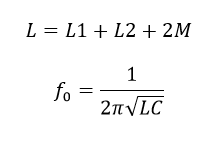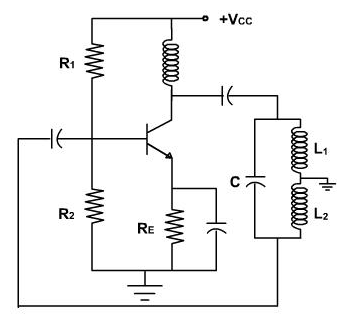# Hartley Oscillator Calculator

This CalcTown calculator calculates the resonant frequency of a Hartley Oscillator Circuit.

The Hartley oscillator is designed for generation of sinusoidal oscillations in the R.F range (20 KHz - 30 MHz). It is very popular and used in radio receivers as a local oscillator.

The circuit diagram of Hartley oscillator (parallel or shunt-fed) using BJT is shown in Figure. It consists of an R-C coupled amplifier using an n-p-n transistor in CE configuration. R1 and R2 are two resistors which form a voltage divider bias to the transistor. A resistor RE is connected in the circuit which stabilizes the circuit against temperature variations. A capacitor CE is connected in parallel with RE, acts as a bypass capacitor and provides a low reactive path to the amplified ac signal. The coupling capacitor CC blocks dc and provides an ac path from the collector to the tank circuit. The L-C feedback network (tank circuit) consists of two inductors L1, and L2 (in series) which are placed across a common capacitor C and the centre of the two inductors is tapped as shown in fig. The feedback network (L1, L2 and C) determines the frequency of oscillation of the oscillator.

#### Result

HzWhere,

C = coupled capacitor

L1 = coupled inductor 1

L2 = coupled inductor 2

M = mutual inductance between the two inductors

L = Equivalent capacitance of the coupled capacitors

f0 = resonant frequency

When the collector supply voltage Vcc is switched on, collector current starts rising and charges the capacitor C. When this capacitor is fully charged, it discharges through coils L1 and L2, setting up damped harmonic oscillations in the tank circuit. The oscillatory current in the tank circuit produces an a.c. voltage across L1 which is applied to the base emitter junction of the transistor and appears in the amplified form in the collector circuit. Feedback of energy from output (collector emitter circuit) to input (base-emitter circuit is) accomplished through auto transformer action. The output of the amplifier is applied across the inductor L1, and the voltage across L2 forms the feedback voltage. The coil L1, is inductively coupled to coil L2, and the combination acts as an auto-transformer. This energy supplied to the tank circuit overcomes the losses occurring in it. Consequently the oscillations are sustained in the circuit.

The energy supplied to the tank circuit is in phase with the generated oscillations. The phase difference between the voltages across L1 and that across L2 is always 180° because the centre of the two is grounded. A further phase of 180° is introduced between the input and output voltages by the transistor itself. Thus the total phase shift becomes 3600 (or zero), thereby making the feedback positive or regenerative which is essential for oscillations. So continuous undamped oscillations are obtained.